# Creating Fractals - MyopicBadger Fractals

## Transcription

Creating Fractals - MyopicBadger Fractals
```Creating Fractals
Graeme Rockhill
January 19, 2015
Contents
1 A Brief Introduction
1.1 Intent . . . . . . . . . . . . . . . . . . . . . . . . . . . . . . . . . . . . . . . . .
1.2 Assumptions . . . . . . . . . . . . . . . . . . . . . . . . . . . . . . . . . . . . . .
1.3 Software . . . . . . . . . . . . . . . . . . . . . . . . . . . . . . . . . . . . . . . .
2 The
2.1
2.2
2.3
2
2
2
2
basics
4
My first fractal . . . . . . . . . . . . . . . . . . . . . . . . . . . . . . . . . . . . 4
Rendering . . . . . . . . . . . . . . . . . . . . . . . . . . . . . . . . . . . . . . . 11
Post-processing . . . . . . . . . . . . . . . . . . . . . . . . . . . . . . . . . . . . 14
3 Some (slightly) advanced
3.1 Final Transforms . . .
3.2 Batch Rendering . . .
3.3 Animation . . . . . . .
techniques
. . . . . . . . . . . . . . . . . . . . . . . . . . . . . . . .
. . . . . . . . . . . . . . . . . . . . . . . . . . . . . . . .
. . . . . . . . . . . . . . . . . . . . . . . . . . . . . . . .
15
15
15
16
4 Displaying your fractals
17
4.1 ImageMagick Montages . . . . . . . . . . . . . . . . . . . . . . . . . . . . . . . . 17
4.2 Using Python to generate HTML galleries . . . . . . . . . . . . . . . . . . . . . 18
1
Chapter 1
A Brief Introduction
1.1
Intent
I’ve been promising myself I’ll write a simple guide to making fractals for a while now, with
the intention of putting it on my website (http://www.myopicbadger.co.uk) to explain the
process behind all the pictures up there. To the surprise of absolutely nobody acquainted with
the volume of hare-brained ideas I have, it’s taken me quite a long time to get round to actually
writing it.
I intend to target this document towards those with no specific knowledge of fractals, beyond
having seen the website, and thinking that they’d like to make their own images like the ones
on there.
1.2
Assumptions
This guide attempts to present a practical, hands-on approach to creating fractal flames using
Apophysis and some supporting tools. If your goal is to create interesting images and learn
to use some handy bits of software, this guide might be for you. If you want to gain an
understanding of the theory behind fractal flames, you will find far better resources elsewhere.
This guide assumes that you’re running a version of Windows that’s reasonably recent (I use
Windows 7 on my main computer, but there’s no reason this guide shouldn’t be fully compatible
with Vista and up) and that you have the ability to install software.
1.3
Software
The software that I use to generate fractals will be introduced properly as it comes up in the
guide. However, I have compiled a list of the core programs involved, on the off-chance that
it’s useful.
• Apophysis 7x (http://apophysis-7x.org/download) - The fractal flame designer at the
heart of this guide.
• GIMP (http://www.gimp.org/) - An open-source alternative to Adobe Photoshop.
In addition to these core programs, I also find the following extremely useful as part of the
process of assembling a collection of fractals into a gallery:
2
CHAPTER 1. A BRIEF INTRODUCTION
3
• ImageMagick (http://www.imagemagick.org/) - A command-line tool for performing
image manipulation. Extremely useful for working with lots of images at once.
• FFmpeg (https://www.ffmpeg.org/) or Libav (https://libav.org/index.html) - A
command-line tool for working with video. Libav is a fork of FFmpeg that came into
being as a result of a falling out between factions in the FFmpeg project. As far as
end users are concerned, they’re basically the same thing, and equivalent commands are
presented for both applications where used in this document.
• Python (https://www.python.org/) - A programming language that’s easy to learn and
useful for scripting.
• Notepad++ (http://notepad-plus-plus.org/) - A source-code editor for Windows
with good support for a variety of languages, including Python.
Chapter 2
The basics
This chapter assumes that you have Apophysis 7x installed, if you do not, it can be downloaded
from www.apophysis-7x.org. Download and install it before continuing with the tutorial.
2.1
My first fractal
Upon opening Apophysis, you should see a window similar to Figure 2.1. Note that several
random fractals have been created for you already. You could base your first fractal off one of
those, if you really wanted to, but for the sake of covering the setup process, this guide will
assume you are creating one from scratch.
Figure 2.1: The main window of Apophysis
Go to File-New (or press Ctrl+N), and you will be presented with a series of preset templates, similar to Figure 2.2. I find these more useful than the random batch, as the presets
4
CHAPTER 2. THE BASICS
5
are known-good fractals, which I can then make more interesting/complex. When I use these,
I tend to use the “plastic” preset – the spherical and julian fractals can be interesting as well,
but the tile effect is easily created by hand, and I dislike the way it resizes the proportions of
the frame.
Figure 2.2: Templates for a new Flame.
For this tutorial, select “Blank Flame” and press “Ok”. When you do, the randomly
generated flame will be replaced with a black screen with faint square in the middle. Obviously,
this isn’t very interesting. Nevertheless, before we remedy that, there’s a little bit of setup it’s
worth doing first.
Figure 2.3: Adjustments, highlighted red.
Select the Adjustments button on the toolbar. A window similar to Figure 2.4 should
appear.
The bulk of the first-page settings on the adjustments panel are fairly self-explanatory.
Depth Blur, Pitch, Yaw, height and Perspective are mostly relevant in the context of 3D fractals
(more about that later). X-Position, Y-Position and Rotation all do exactly what you’d expect.
A quick note on Scale and Zoom, however. These are not the same thing. In the context of
designing fractals, you want to use scale, rather than zoom (whether via the adjustments or the
CHAPTER 2. THE BASICS
6
Figure 2.4: The Adjustments panel
main window toolbar). To see why, first try tripling the scale of the image to 10, then return it
to what it was, and increase the zoom to 3, and compare the time it takes for the main window
to update. Before you leave this tab, set the scale to 15, and ensure that the zoom is reset to
0.
Next, head to the rendering tab, and increase the gamma slider to four or five. This will
ensure the resulting fractal doesn’t appear annoyingly dim. The gradient tab is a bit more
interesting.
Figure 2.5: The Gradient Adjustment Panel
Ultimately, this is one of those panels where you should fiddle with all the options to see
what they do, once you have a fractal you’re reasonably happy with. The two elements worth
mentioning now are the preset button, which selects a random colour palette from the default
list, and the dropdown box, which contains a vast array of presets to chose from. I’m far from
an authority on colour schemes, but as a rule, you want a palette that contains a couple of
complementary colours, including one very light and one very dark shade.
Next up, the Image Size tab. If the Gradients adjustment is a screen to play with, the image
size adjustments screen is one to set once, and only ever visit to ensure it stays set.
Here you need to know what dimensions you intend for the final render. The values you
enter in the height and width boxes should be in the aspect ratio as your final render. In
CHAPTER 2. THE BASICS
7
Figure 2.6: The Image Size Adjustment Panel
my case, I render most of my fractals for use as desktop wallpapers on my 1920x1200 (16:10)
computer monitor, so I will set the width and height to 960x600 – smaller doesn’t matter, the
aim is to ensure that what you see while you design it matches what you see once you render
it. If your final render is going to be Full HD (1920x1080 or 16:9) then a good choice would be
960x540. Once you’ve chosen a height and width you’re happy with, press the floppy disk icon
next to one of the buttons in the middle divide, then press the corresponding button. Whenever
you create a new fractal, you will probably have to reset the width and height. We’re going to
ignore the curves tab for the time being, so close the adjustments panel and return to the main
window.
From here, there are a couple of ways to proceed, either carefully, using the editor, or
quickly, using mutations.
Figure 2.7: Editor, highlighted red. Mutations, highlighted blue.
Whichever you chose, you’ll likely end up using the Editor, so we’ll cover Mutations first.
Open the Mutations panel (the toolbar button is highlighted in blue on Figure 2.7) and pick
one of the nine options (Figure 2.8). You can repeat this as many times as you feel happy with.
If you went via the mutation route, when you open the editor window (Figure 2.9) you will
see three or more triangles here. Otherwise, you’ll see a single red triangle. You’ll notice that
the entry for “linear” in the bottom right will be 1. Right-click on the triangle, and select ”new
transform” from the context menu.
This will create a yellow triangle directly on top of the red one. From here, you should
experiment with moving the triangles around, changing their scales and proportions and so on.
As a general rule when creating fractals, adjusting things roughly using the mouse produces
more interesting results than exactly.
For the sake of producing a simple fractal for this tutorial, I suggest rotating the yellow
triangle 45 degrees counter-clockwise, so that the dotted face is facing upwards. You can do
this using the smooth curve on the ”triangle” tab of the right hand panel, (figure 2.10) or by
dragging either of the solid lines of the triangle. Dragging the dotted edge will affect the scale
of the triangle.
If you look in the main apophysis window, the simple transform I’ve described will not
look very impressive - you should see a faintly coloured blob, which faintly resembles an eightpointed star. If you were to increase the density of the preview in the main window, or rendered
CHAPTER 2. THE BASICS
8
Figure 2.8: The Mutations Panel
Figure 2.9: The Editor Panel
CHAPTER 2. THE BASICS
9
the fractal now, you would see that the fractal consists of two semi-transparent squares, one
rotated 45-degrees in relation to the other. This is the essence of the linear transformation.
Figure 2.10: The Editor Triangle Panel
One thing we can do to make the fractal more interesting is tweak the colour. To do this,
we need to go to the “colours” tab in the right hand window. If you select each triangle in
turn, you will see the transform colour for both is set to the same value. Set the transform
colour for the yellow triangle to 1. Depending on what gradient colours you chose earlier (see
figure 2.5) you may see the difference immediately, but you probably won’t – many of the built
in gradients have the same colour at 0 and 1, allowing them to be tiled smoothly. Don’t worry
too much about this, the purpose of the exercise is to ensure that as we make the fractal more
complicated, we get the full range of the gradient, rather than just one end.
The other thing we can do to make the fractal more interesting is start altering the transforms themselves. Go to the variations tab in the editor, and make sure you select the first (red)
transform. Find the row labelled “linear” and decrease that to 0, then find the row labelled
“spherical” and set that to 1. Then go to the second, yellow transform, find the row labelled
“linear” and decrease that to 0, then find the row labelled “cylinder” and set that to about 0.5.
Once you’ve done that, the main preview window should look something like figure 2.11.
This is a bit more interesting, I think you’ll agree.
As a general rule, when creating fractals, every subsequent transform you add beyond the
second has a progressively smaller impact on the general shape of the resulting fractal. If, for
example, you were to add a third transform, with linear set to 0, but julia3Dz set to about 0.25,
the basic shape of the fractal would stay the same, but the detail would change substantially.
See figure 2.12 for an example.
CHAPTER 2. THE BASICS
Figure 2.11: Getting a bit more interesting.
Figure 2.12: 0.25 julia in transform three.
10
CHAPTER 2. THE BASICS
11
Experimentation is the best way to figure out which transforms produce results you like.
When you’re happy, press Ctrl+S to bring up the “Save Parameters” dialog (Figure 2.13)
Figure 2.13: The “Save Parameters” dialog
Note the presence of both “Destination” and “Name” fields. The .flame file format can
contain multiple fractals, so long as they have different names. See section 3.2 for more explanation about why this is useful. For now, all you need to remember is that you should always
save using a unique name, even if it’s just adding the date or time to the end of the default
suggestion (or a number).
2.2
Rendering
To begin the process of rendering a fractal, you first need a fractal you’re happy with – for the
sake of this tutorial, I will assume you have saved your fractal from section 2.1.
The first step is to open your flame file – to do this, press Ctrl+O, and navigate to it in
the browse window. The right hand window should update with the list of flames contained in
the file. Click on the one you want to render, and the main preview window should update to
contain the flame. Then, go to the main toolbar, and select the single cogwheel icon.
This will open up the Render Dialog (figure 2.14). Many of the options on here are fairly
straightforward. The destination is the file that the result will be saved to, height and width
are exactly what you’d expect. The suffix of the destination file determines the output format.
PNG allows transparency, and we’re going to have to do some post-processing either way, so
you might as well keep your options open on the brackground. The quality settings might
require a larger degree of explanation, however.
Density, as the name implies, relates to the density of the fractal. Increasing the density of
the fractal increases the time it takes to render more or less linearly. For reference, the preview
window in apophysis (and thus figures 2.11 and 2.12) render at a density of 35, and the rendered
fractal in figure 2.15 was rendered at 1000. In general, I render fractals at 1000 density – 500
often produces acceptable results when the fractal has hard edges, but will appear “spotty” in
smooth areas, and higher than 2000 doesn’t produce results worth the extra time.
Filter Radius is essentially the level of smoothing applied to the final result. The default 0.4
appears a bit sharp for my taste, I prefer it increased to one, although to some extent it depends
on the kind of fractals you prefer to create. It doesn’t significantly increase the length of time
it take to render – this occurs in the final stages of the process (if you check the “post-process
after rendering” you can change a couple of the settings in this before saving to file) and should
only take a few seconds.
Oversample causes the image to be rendered at several times the size of the final file, then
smoothed down. This does increase render time somewhat, and significantly increases the
CHAPTER 2. THE BASICS
12
Figure 2.14: The Render Window
memory requirements.
The other extremely significant setting related to rendering is the number of processor cores
being used by the render. For reasons unclear to me, you cannot change this in the render
dialog, and must instead enable it in the main application settings. Multiple threads will
significantly shorten the time it takes to render the fractal, but remember that using all the
cores in your computer will make it extremely sluggish until the render is complete. I tend to
render large batches overnight for this reason.
Once you’re happy with the settings, press the button labelled “Start” to begin rendering.
The render window will automatically switch to the “output” tab, which will contain more
information about the progress of the render.
CHAPTER 2. THE BASICS
13
Figure 2.15: The fractal from figure 2.11 rendered at density=1000, filter radius=1, oversample=4, 960x600 and scaled to fit the page
Figure 2.16: The fractal from figure 2.12 rendered at density=1000, filter radius=1, oversample=4, 960x600 and scaled to fit the page
CHAPTER 2. THE BASICS
2.3
14
Post-processing
Assuming you’ve been following the proceeding chapters, you will now have a high quality .png
image file of your fractal. You could use that image file as is. However, some programs will
struggle with transparent PNG files, and the file apophysis is far larger than it necessarily needs
to be.
Both of these things can be rectified relatively simply. GIMP (or Photoshop) is the obvious
tool to do this – the process of adding a new, solid black layer and exporting the altered image
as a JPEG image is trivial, and not covered in this guide.
Obviously, this process will become incredibly tedious when performed more than once
or twice. Fortunately there’s a simple alternative. ImageMagick (http://www.imagemagick.
org/) is a command line image-editing tool. If you’re not familiar with the command-line, do
not be intimidated – it’s pretty straightforward to use.
Open the command-line in the directory where your images are located. The simplest way
to do this is to right-click on the folder in windows explorer while holding the shift key. This
opens the extended context menu, which will have the option “open command window here”
near the top of the list.
From there you would simply type the following into the command prompt:
c o m p o s i t e −compose Dst Over − t i l e p a t t e r n :GRAY0 i n f i l e . png o u t f i l e .
,→ j p g
This will add a black background to infile.png and save the resulting image to outfile.jpg
– note the change in extensions, the resulting JPEG image will be much smaller that the input
PNG. For reference, the fractal in Figure 2.16 came out of Apophysis as a 1.5 MB PNG file,
but became an 81 KB JPEG without a visible loss of quality. You can use a white background
instead of a black one by replacing GRAY0 with GRAY100. A full list of supported colours can
be found here: http://www.imagemagick.org/script/color.php#color_names
Chapter 3
Some (slightly) advanced techniques
3.1
Final Transforms
Final transforms are a good way to create striking variations of already polished fractals. To
apply a final transform to a fractal, open the editor window and click the final transform button
(Figure 3.1).
Figure 3.1: The Final Transform button
This will create a white triangle that can be edited exactly as per the other transforms.
The final transform represents a purer expression of the underlying variation, as it applies to
the entire, final fractal.
3.2
Batch Rendering
Batch Rendering is the logical continuation of the knowledge that a .flame file can contain
multiple fractals (stated towards the end of section 2.1). Starting a batch render is simply a
matter of opening a flame file containing multiple fractals and pressing the icon for “Render all
flames” (the double cogwheel icon located next to the single render button).
This will open a window identical to Figure 2.14. The destination file will be the first file
to be rendered, but settings chosen will be applied to all the fractals being rendered.
Obviously, manually post-processing every image will be incredibly tedious. Fortunately,
the command-line is again our saviour. Many imagemagick commands have their own built in
batch processing, unfortunately, composite likes to be a bit awkward. Instead, we’re going to
create a batch file.
Right-click in your folder, and select “New Text Document”. Rename it something like
“black-and-white-backgrounds.bat” and open it in notepad.
Add the code below to the file and save it.
f o r f i l e s /m ∗ . png / c ”CMD / c c o m p o s i t e −compose Dst Over − t i l e
,→ p a t t e r n :GRAY0 @FILE @FNAME−b l a c k . j p g ”
f o r f i l e s /m ∗ . png / c ”CMD / c c o m p o s i t e −compose Dst Over − t i l e
,→ p a t t e r n : GRAY100 @FILE @FNAME−white . j p g ”
15
CHAPTER 3. SOME (SLIGHTLY) ADVANCED TECHNIQUES
16
This code finds every .png file in the folder where the batch file is run, and runs the composite
command from section 2.3 with that file as the subject, creating an output file with a black
background, then does the same creating an image with a white background.
You can run this batch file by double clicking it in windows explorer, or by typing the name
of the batch file in the command prompt while working in the folder.
3.3
Animation
Creating animated fractals is a bit convoluted. The basic premise of the process is that you
batch render a sequence of fractals, then run the output through ffmpeg or libav using the
following command to stitch the images (in this case assumed to be named ”sequence (1).jpg”,
”sequence (2).jpg”, etc) into a single video file.
ffmpeg −f image2 −f r a m e r a t e 30 −i ” s e q u e n c e (%d ) . j p g ” out . mp4
or, if you have installed libav:
avconv −f image2 −f r a m e r a t e 30 −i ” s e q u e n c e (%d ) . j p g ” out . mp4
In previous versions of apophysis, it was possible to run scripts that would interpolate
between manually created keyframes, making it much easier to create animations. However,
the scripting engine has been removed from more recent versions (there were licensing issues, I
believe) and I have yet to find a suitable replacement. When I do, I will update this document
accordingly.
Chapter 4
Displaying your fractals
4.1
ImageMagick Montages
These are what I used to use for my fractals before I started working with python and HTML.
I’ve long since lost the command I used, but if you want to try this, the official documentation
is the place to start: http://www.imagemagick.org/Usage/montage/
UPDATE: Since the original version of this document, I’ve actually found most of the
invocations I thought I’d lost.
The basic imagemagick command used to generate the image gallery was this:
montage +l a b e l −frame 5 − t i l e 4x −background #999999 −geometry 250
,→ x250+4+4 ∗ . j p g g a l l e r y . html
This goes through the current directory and creates a montage of every JPEG in the current
directory, along with a HTML file imagemap to each image. This will look something like figure
4.1.
The downside of this approach is the size of the output file. The imagemap is a PNG file
that, in a reasonably large sized gallery, could easily be several megabytes. This is fine for
viewing locally, but over the internet, will take several seconds to download. There are several
ways we could shrink the montage file and still use the generated HTML. Part of the problem is
that PNG is a lossless image format - great for preserving image quality, bad for making small
files. The solution is to convert our PNG into a JPEG. You could do this manually in GIMP,
of course, but that’s boring. Instead, we could do it with our montage command. Running
it again with the output changed to JPEG will generate an identical image that will be much
smaller.
montage +l a b e l −frame 5 − t i l e 4x −background #999999 −geometry 250
,→ x250+4+4 ∗ . j p g g a l l e r y . j p g
However, It might be faster to convert the PNG directly, rather than regenerating the
montage. This can be done simply with the imagemagic convert command:
c o n v e r t g a l l e r y . png g a l l e r y . j p g
Then you simply open the HTML file in a text editor, and find-and-replace “gallery.png”
with “gallery.jpg”.
17
CHAPTER 4. DISPLAYING YOUR FRACTALS
18
Figure 4.1: The output of the montage command
4.2
Using Python to generate HTML galleries
This is the approach I’ve taken with MyopicBadger. The code is a bit of a mess, so I’ve
rewritten the absolute basics, and I leave expanding on it as an exercise for the reader.
All three files are included in the zip. The text of the python script is included below:
http://www.myopicbadger.co.uk/static/documents/builder.zip
import sys , s u b p r o c e s s , os , s h u t i l , d a t e t i m e
d e f debug ( t e x t ) :
print ( text )
def createGallery () :
c u r r e n t l o c a t i o n = os . getcwd ( )
galleryName = os . path . basename ( os . path . normpath (
,→ c u r r e n t l o c a t i o n ) )
debug ( ” G a l l e r y Name : ” + galleryName )
debug ( ” . . . running i n ”+c u r r e n t l o c a t i o n )
CHAPTER 4. DISPLAYING YOUR FRACTALS
19
d o S l o w S t u f f = True
#d o S l o w S t u f f = F a l s e
i f doSlowStuff :
# t h i s s t u f f i s q u i t e slow , and we o n l y r e a l l y need
,→ t o do i t once
debug ( ” F u l l r e b u i l d : ”+galleryName )
# d e l e t e the thumbnail folder , i f i t e x i s t s
s h u t i l . rmtree ( ’ thumbs ’ , ” t r u e ” )
# we ’ l l be remaking g a l l e r y . html from s c r a t c h
,→ r e g a r d l e s s
f o r f i l e n a m e i n os . l i s t d i r ( ” . ” ) :
i f f i l e n a m e == ” g a l l e r y . html ” : # or f i l e n a m e
,→ == ” t e x t . t x t ” :
os . remove ( f i l e n a m e )
# make a new f o l d e r i n t h e c u r r e n t f o l d e r f o r t h e
,→ t h u m b n a i l s
debug ( ” . . . c r e a t i n g thumbs s u b f o l d e r ” )
os . mkdir ( ”thumbs” )
debug ( ” . . . made thumbs f o l d e r ” )
# generate the thumnails themselves
s u b p r o c e s s . c a l l ( ” m o g r i f y −format j p g −path thumbs −
,→ thumbnail 250 x250 ∗ . j p g ” )
debug ( ” . . . t h u m n a i l i n g complete ” )
# Now, we ’ l l add some e x i f d a t a f o r t h e images
debug ( ” . . . g e n e r a t i n g EXIF” )
# open our t e m p l a t e f i l e f o r our e x i f d a t a . The
,→ t e m p l a t e f i l e i s p r e t t y s e l f −e x p l a n a t o r y
f 1 = open ( ’ t e x t −t e m p l a t e . t x t ’ , ’ r ’ )
# create a modified version of the e x i f t i l e that
,→ has t h e c u r r e n t d a t e i n i t . 1
f 2 = open ( ’ t e x t . t x t ’ , ’w ’ )
for l i n e in f1 :
l i n e = l i n e . r e p l a c e ( ” ! now ! ” , d a t e t i m e .
,→ d a t e t i m e . now ( ) . s t r f t i m e ( ”%Y−%m−%d %H:%
,→ M:%S.% f ” ) )
f2 . write ( l i n e )
f1 . close ()
f2 . close ()
# now , w r i t e t h e e x i f t e x t f i l e i n t o t h e images .
debug ( ” . . . stamping EXIF” )
s u b p r o c e s s . c a l l ( ” m o g r i f y − p r o f i l e 8BIMTEXT: t e x t . t x t
,→ ∗ . j p g ” )
CHAPTER 4. DISPLAYING YOUR FRACTALS
20
s u b p r o c e s s . c a l l ( ” m o g r i f y − p r o f i l e 8BIMTEXT: t e x t . t x t
,→ thumbs / ∗ . j p g ” )
debug ( ” . . . EXIF stamped ” )
else :
debug ( ” . . . s k i p p i n g image r e g e n e r a t i o n ” )
#s p e c i f y t h e code f o r each i n d i v i d u a l image
g r i d C e l l = ’<d i v c l a s s =”emptySpace ” data−backdrop =”! backdrop
,→ !”>< d i v c l a s s =” f l o a t e d i m g img−thumbnail”><a h r e f =”!
,→ f u l l i m a g e ! ” data−l i g h t b o x =” g a l l e r y ”><img s r c =”!
,→ thumbimage ! ” a l t =”! f u l l i m a g e ! ” ></a><br />[<a h r e f =”!
,→ f u l l i m a g e !”> F u l l s i z e </a>]</div ></div> ’
# make an a r r a y o f e v e r y f i l e i n t h e d i r e c t o r y
f u l l i m a g e f i l e s = [ f f o r f i n os . l i s t d i r ( ’ . ’ ) i f os . path .
,→ i s f i l e ( f ) ]
# l o o p t h r o u g h e v e r y image , and add them t o t h e g r i d , i f
,→ t h e y have t h e e x t e n s i o n . j p g
g r i d B o x e s = ””
for f i l e in f u l l i m a g e f i l e s :
i f s t r ( f i l e ) . f i n d ( ” . j p g ” ) > −1:
backdrop = ”UNKNOWN”
i f s t r ( f i l e ) . f i n d ( ”−b l a c k ” ) > −1:
backdrop = ”BLACK”
i f s t r ( f i l e ) . f i n d ( ”−white ” ) > −1:
backdrop = ”WHITE”
g r i d B o x e s += g r i d C e l l . r e p l a c e ( ” ! f u l l i m a g e ! ” ,
,→ f i l e ) . r e p l a c e ( ” ! thumbimage ! ” , ”thumbs
,→ /”+ f i l e ) . r e p l a c e ( ” ! backdrop ! ” ,
,→ backdrop )
# open t h e g a l l e r y t e m p l a t e and t h e temporary g a l l e r y
f 1 = open ( ’ g a l l e r y −t e m p l a t e . html ’ , ’ r ’ )
f 2 = open ( ’ g a l l e r y . html . tmp ’ , ’w ’ )
# go t h r o u g h t h e t e m p l a t e , r e p l a c i n g any keywords w i t h t h e i r
,→ r e s p e c t i v e c o n t e n t , and s a v i n g i t t o t h e temp f i l e .
for l i n e in f1 :
l i n e = l i n e . r e p l a c e ( ’ ! brand ! ’ , galleryName )
l i n e = l i n e . r e p l a c e ( ’ ! t i t l e ! ’ , galleryName )
l i n e = l i n e . replace ( ’ ! gridboxes ! ’ , gridBoxes )
l i n e = l i n e . r e p l a c e ( ” ! now ! ” , d a t e t i m e . d a t e t i m e . now ( )
,→ . s t r f t i m e ( ”%Y−%m−%d %H:%M:%S.% f ” ) )
f2 . write ( lin e )
f1 . close ()
f2 . close ()
debug ( ” . . . r e p l a c e complete ” )
CHAPTER 4. DISPLAYING YOUR FRACTALS
# do some t i d y i n g up
f o r f i l e n a m e i n os . l i s t d i r ( ” . ” ) :
i f f i l e n a m e == ” g a l l e r y . html ” :
os . remove ( f i l e n a m e )
debug ( ” . . . removed o l d g a l l e r y . html ” )
i f f i l e n a m e == ” g a l l e r y −t e m p l a t e . html ” :
#os . remove ( f i l e n a m e )
debug ( ” . . . removed t e m p l a t e . html ” )
i f f i l e n a m e == ” g a l l e r y . html . tmp” :
os . rename ( f i l e n a m e , ” g a l l e r y . html ” )
debug ( ” . . . renamed tmp g a l l e r y ” )
i f f i l e n a m e == ” t e x t . t x t ” :
#os . remove ( f i l e n a m e )
debug ( ” . . . removed l o c a l copy o f IPTC f i l e ” )
debug ( ” . . . F i l e o p e r a t i o n s complete ” )
if
name
== ’ m a i n ’ :
# b e i n g e x e c u t e d as s c r i p t
createGallery ()
21
```

### protint 751 update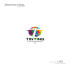More information

### Fractals, Dynamical Systems and Chaos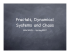More information

### Slides of the fifth lecture (Fractals and multifractals, 4739KB)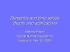More information

### Exploring the Effect of Direction on Vector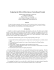More information

### (Microsoft PowerPoint - La complessit\340 della realt\340 biologica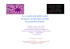More information

### Fractals and 3D printing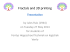More information

### Chapter 8 Fractal properties of plants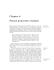More information

### Fractal Pack- educator`s guide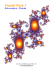More information

### Perceptual and Physiological Responses to the Visual Complexity of R.P. Taylor,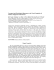More information

### A short history of fractal-Cantorian space-time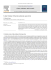More information

### Verdict - cadpoint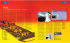More information

### Render layers in Maya and in post processing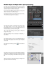More information

### reviewed paper A Multi-Scale (Multi-Fractal) Approach for a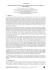More information

### Analyzing the Terrorist Social Networks with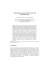More information

### Introduction - Ultra Fractal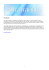More information